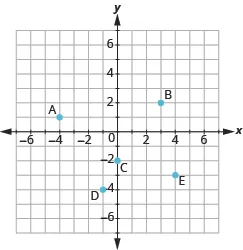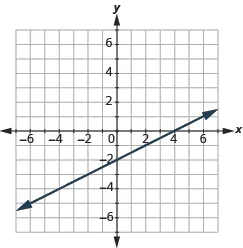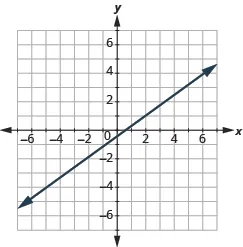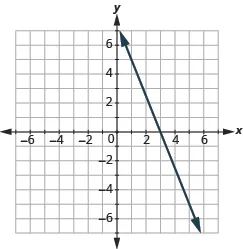Prealgebra 2e

# Practice Test

Prealgebra 2ePractice Test

### Practice Test

339.

Plot and label these points:

1. $(2,5)(2,5)$
2. $(−1,−3)(−1,−3)$
3. $(−4,0)(−4,0)$
4. $(3,−5)(3,−5)$
5. $(−2,1)(−2,1)$
340.

Name the ordered pair for each point shown.341.

Find the $x-interceptx-intercept$ and $y-intercepty-intercept$ on the line shown.342.

Find the $x-interceptx-intercept$ and $y-intercepty-intercept$ of the equation $3x−y=6.3x−y=6.$

343.

Is $(1,3)(1,3)$ a solution to the equation $x+4y=12?x+4y=12?$ How do you know?

344.

Complete the table to find four solutions to the equation $y=−x+1.y=−x+1.$

 $xx$ $yy$ $(x,y)(x,y)$ $00$ $11$ $33$ $−2−2$
345.

Complete the table to find three solutions to the equation $4x+y=84x+y=8$

 $xx$ $yy$ $(x,y)(x,y)$ $00$ $00$ $33$

In the following exercises, find three solutions to each equation and then graph each line.

346.

$y = −3 x y = −3 x$

347.

$2 x + 3 y = −6 2 x + 3 y = −6$

In the following exercises, find the slope of each line.

348.349.350.

Use the slope formula to find the slope of the line between $(0,−4)(0,−4)$ and $(5,2).(5,2).$

351.

Find the slope of the line $y=2.y=2.$

352.

Graph the line passing through $(1,1)(1,1)$ with slope $m=32.m=32.$

353.

A bicycle route climbs $2020$ feet for $1,0001,000$ feet of horizontal distance. What is the slope of the route?

Order a print copy

As an Amazon Associate we earn from qualifying purchases.